# 八、降维

## 维数灾难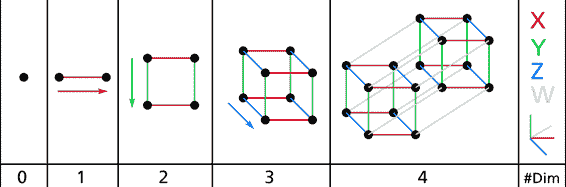## 降维的主要方法

### 投影（Projection）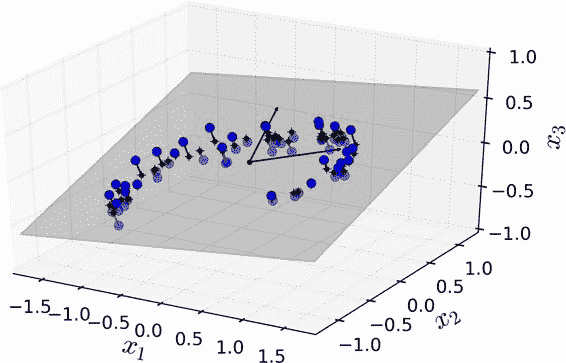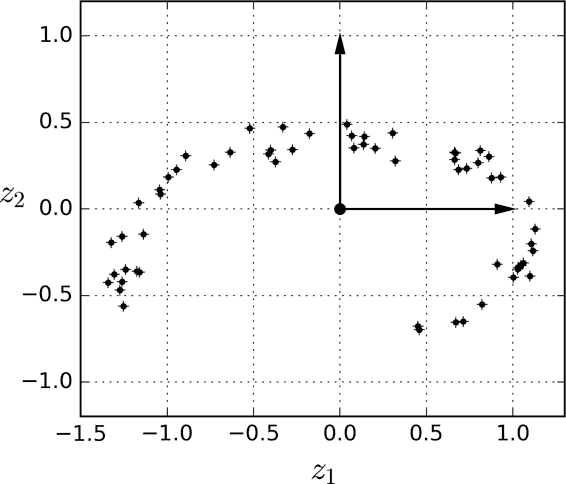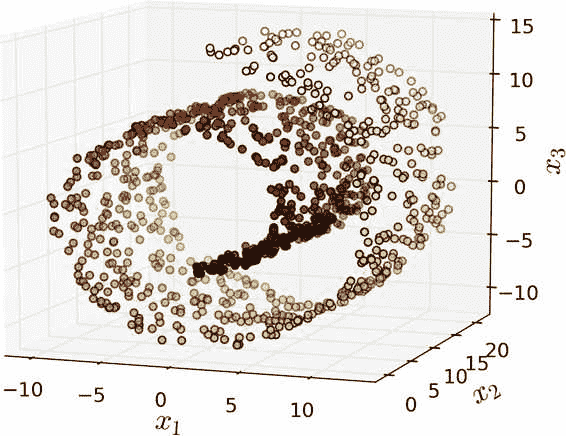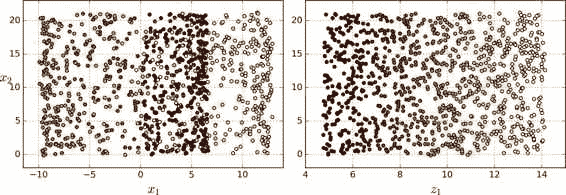### 流形学习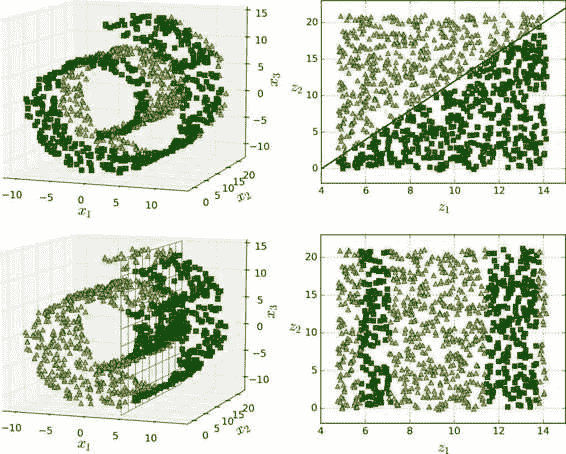## 主成分分析（PCA）

### 保留（最大）方差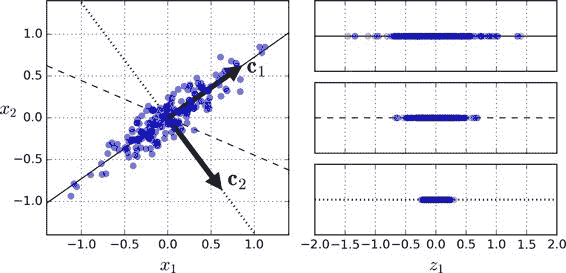### 主成分（Principle Componets）

PCA 寻找训练集中可获得最大方差的轴。在图 8-7 中，它是一条实线。它还发现了一个与第一个轴正交的第二个轴，选择它可以获得最大的残差。在这个 2D 例子中，没有选择：就只有这条点线。但如果在一个更高维的数据集中，PCA 也可以找到与前两个轴正交的第三个轴，以及与数据集中维数相同的第四个轴，第五个轴等。 定义第i个轴的单位向量被称为第i个主成分（PC）。在图 8-7 中，第一个 PC 是c1，第二个 PC 是c2。在图 8-2 中，前两个 PC 用平面中的正交箭头表示，第三个 PC 与上述 PC 形成的平面正交（指向上或下）。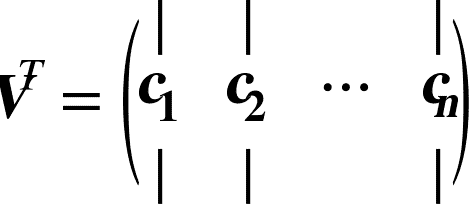X_centered=X-X.mean(axis=0)
U,s,V=np.linalg.svd(X_centered)
c1=V.T[:,0]
c2=V.T[:,1]


### 投影到d维空间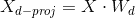W2=V.T[:,:2]
X2D=X_centered.dot(W2)


### 使用 Scikit-Learn

Scikit-Learn 的 PCA 类使用 SVD 分解来实现，就像我们之前做的那样。以下代码应用 PCA 将数据集的维度降至两维（请注意，它会自动处理数据的中心化）：

from sklearn.decomposition import PCA

pca=PCA(n_components=2)
X2D=pca.fit_transform(X)


### 方差解释率（Explained Variance Ratio）

>>> print(pca.explained_variance_ratio_)
array([0.84248607, 0.14631839])


### 选择正确的维度

pca=PCA()
pac.fit(X)
cumsum=np.cumsum(pca.explained_variance_ratio_)
d=np.argmax(cumsum>=0.95)+1


pca=PCA(n_components=0.95)
X_reduced=pca.fit_transform(X)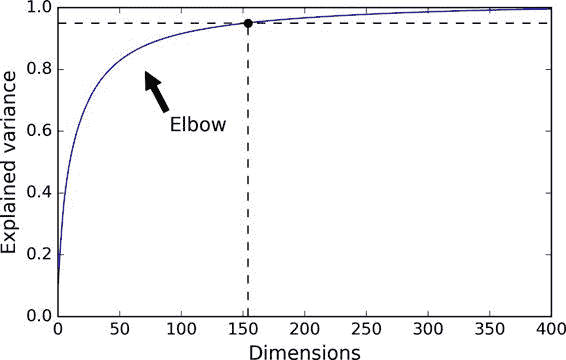### PCA 压缩

pca=PCA(n_components=154)
X_mnist_reduced=pca.fit_transform(X_mnist)
X_mnist_recovered=pca.inverse_transform(X_mnist_reduced)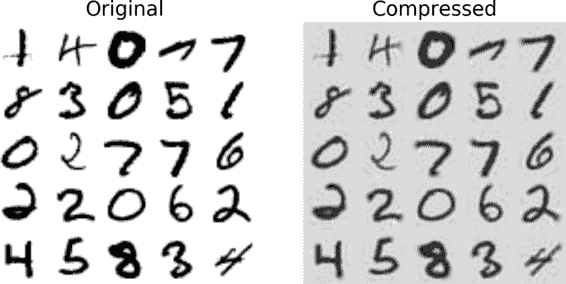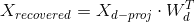### 增量 PCA（Incremental PCA）

from sklearn.decomposition import IncrementalPCA

n_batches=100
inc_pca=IncrementalPCA(n_components=154)
for X_batch in np.array_spplit(X_mnist,n_batches):
inc_pca.partial_fit(X_batch)
X_mnist_reduced=inc_pca.transform(X_mnist)


X_mm=np.memmap(filename,dtype='float32',mode='readonly',shape=(m,n))
batch_size=m//n_batches
inc_pca=IncrementalPCA(n_components=154,batch_size=batch_size)
inc_pca.fit(X_mm)


### 随机 PCA（Randomized PCA）

Scikit-Learn 提供了另一种执行 PCA 的选择，称为随机 PCA。这是一种随机算法，可以快速找到前d个主成分的近似值。它的计算复杂度是O(m × d^2) + O(d^3)，而不是O(m × n^2) + O(n^3)，所以当d远小于n时，它比之前的算法快得多。

rnd_pca=PCA(n_components=154,svd_solver='randomized')
X_reduced=rnd_pca.fit_transform(X_mnist)


## 核 PCA（Kernel PCA）

from sklearn.decomposition import KernelPCA

rbf_pca=KernelPCA(n_components=2,kernel='rbf',gamma=0.04)
X_reduced=rbf_pca.fit_transform(X)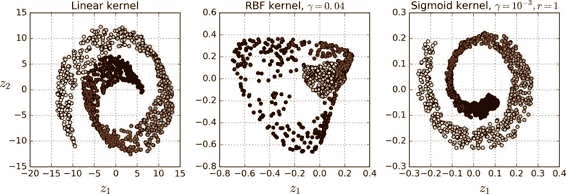### 选择一种核并调整超参数

from sklearn.model_selection import GridSearchCV
from sklearn.linear_model import LogisticRegression
from sklearn.pipeline import Pipeline

clf = Pipeline([
("kpca", KernelPCA(n_components=2)),
("log_reg", LogisticRegression())
])
param_grid = [{
"kpca__gamma": np.linspace(0.03, 0.05, 10),
"kpca__kernel": ["rbf", "sigmoid"]
}]
grid_search = GridSearchCV(clf, param_grid, cv=3)
grid_search.fit(X, y)


>>> print(grid_search.best_params_)
{'kpca__gamma': 0.043333333333333335, 'kpca__kernel': 'rbf'}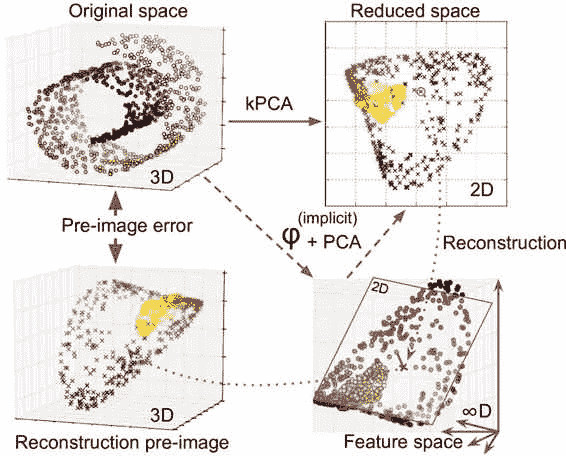rbf_pca = KernelPCA(n_components = 2, kernel="rbf", gamma=0.0433,fit_inverse_transform=True)
X_reduced = rbf_pca.fit_transform(X)
X_preimage = rbf_pca.inverse_transform(X_reduced)


>>> from sklearn.metrics import mean_squared_error
>>> mean_squared_error(X, X_preimage) 32.786308795766132


### LLE

from sklearn.manifold import LocallyLinearEmbedding

lle=LocallyLinearEmbedding(n_components=2,n_neighbors=10)
X_reduced=lle.fit_transform(X)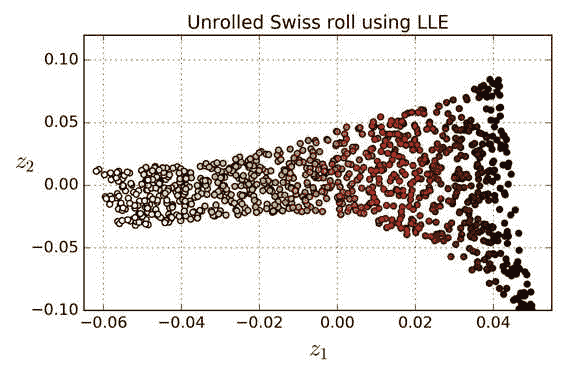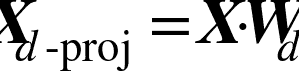Scikit-Learn 的 LLE 实现具有如下的计算复杂度：查找k个最近邻为O(m log(m) n log(k))，优化权重为O(m n k^3)，建立低维表示为O(d m^2)。不幸的是，最后一项m^2使得这个算法在处理大数据集的时候表现较差。

## 其他降维方法

• 多维缩放（MDS）在尝试保持实例之间距离的同时降低了维度（参见图 8-13）
• Isomap 通过将每个实例连接到最近的邻居来创建图形，然后在尝试保持实例之间的测地距离时降低维度。
• t-分布随机邻域嵌入（t-Distributed Stochastic Neighbor Embedding，t-SNE）可以用于降低维​​度，同时试图保持相似的实例临近并将不相似的实例分开。它主要用于可视化，尤其是用于可视化高维空间中的实例（例如，可以将 MNIST 图像降维到 2D 可视化）。
• 线性判别分析（Linear Discriminant Analysis，LDA）实际上是一种分类算法，但在训练过程中，它会学习类之间最有区别的轴，然后使用这些轴来定义用于投影数据的超平面。LDA 的好处是投影会尽可能地保持各个类之间距离，所以在运行另一种分类算法（如 SVM 分类器）之前，LDA 是很好的降维技术。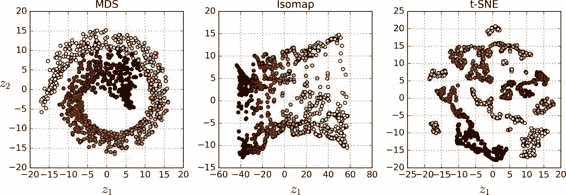## 练习

1. 减少数据集维度的主要动机是什么？主要缺点是什么？
2. 什么是维度爆炸？
3. 一旦对某数据集降维，我们可能恢复它吗？如果可以，怎样做才能恢复？如果不可以，为什么？
4. PCA 可以用于降低一个高度非线性对数据集吗？
5. 假设你对一个 1000 维的数据集应用 PCA，同时设置方差解释率为 95%，你的最终数据集将会有多少维？
6. 在什么情况下你会使用普通的 PCA，增量 PCA，随机 PCA 和核 PCA？
7. 你该如何评价你的降维算法在你数据集上的表现？
8. 将两个不同的降维算法串联使用有意义吗？
9. 加载 MNIST 数据集（在第 3 章中介绍），并将其分成一个训练集和一个测试集（将前 60,000 个实例用于训练，其余 10,000 个用于测试）。在数据集上训练一个随机森林分类器，并记录了花费多长时间，然后在测试集上评估模型。接下来，使用 PCA 降低数据集的维度，设置方差解释率为 95%。在降维后的数据集上训练一个新的随机森林分类器，并查看需要多长时间。训练速度更快？接下来评估测试集上的分类器：它与以前的分类器比较起来如何？
10. 使用 t-SNE 将 MNIST 数据集缩减到二维，并使用 Matplotlib 绘制结果图。您可以使用 10 种不同颜色的散点图来表示每个图像的目标类别。或者，您可以在每个实例的位置写入彩色数字，甚至可以绘制数字图像本身的降维版本（如果绘制所有数字，则可视化可能会过于混乱，因此您应该绘制随机样本或只在周围没有其他实例被绘制的情况下绘制）。你将会得到一个分隔良好的的可视化数字集群。尝试使用其他降维算法，如 PCA，LLE 或 MDS，并比较可视化结果。